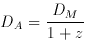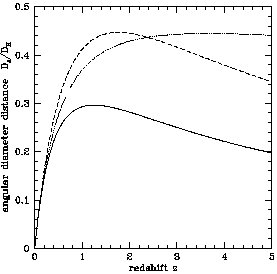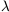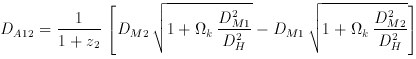### 6. ANGULAR DIAMETER DISTANCE

The angular diameter distance DA is defined as the ratio of an object's physical transverse size to its angular size (in radians). It is used to convert angular separations in telescope images into proper separations at the source. It is famous for not increasing indefinitely as z ->; it turns over at z ~ 1 and thereafter more distant objects actually appear larger in angular size. Angular diameter distance is related to the transverse comoving distance by(17)

(Weinberg 1972, pp. 421-424; Weedman 1986, pp. 65-67; Peebles 1993, pp. 325-327). The angular diameter distance is plotted in Figure 2.Figure 2. The dimensionless angular diameter DA / DH. The three curves are for the three world models, (M,) = (1, 0), solid; (0.05, 0) dotted; and (0.2, 0.8) dashed.

There is also an angular diameter distance DA12 between two objects at redshifts z1 and z2, frequently used in gravitational lensing. It is not found by subtracting the two individual angular diameter distances! The correct formula, fork0, is(18)

where DM1 and DM2 are the transverse comoving distances to z1 and z2, DH is the Hubble distance, andk is the curvature density parameter (Peebles 1993, pp. 336-337). Unfortunately, the above formula is not correct fork < 0 (Phillip Helbig, 1998, private communication).